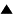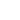# Knowledge

[Node to be completed]

In cybernetics we say that a purposive cybernetic system S has some knowledge if the system S has a model of some part of reality as it is perceived by the system. Informally, a model of some process occurring in a system is a another system which somehow mimicks, or simulates, that process; thus by using the model it becomes possible to know something about the modelled process without actually running it, or predict developments before they actually happen.

The definition of the modeling scheme includes an important assumption of non-interference: that the procedure R (which is a sequence of actions), when it acts on a state w of the world, does not change that state. But we know from quantum mechanics that this assumption, strictly speaking, never holds. When we deal with macroscopic phenomena we can, nevertheless, use the non-interference assumpiton, because the impact of the instruments we use in R on the state w can always be made insignificant. But on the atomic and subatomic levels, we cannot abstract from the action of the means we use to get information about the objects studied.

There is one more non-interference assumption: the modeling procedure M_a imitating the action a does not alter in any way the course of the action, including the final result w_2. Usually, this assumption is easily true; the most salient case is that of an astronomer. His computations, certainly, do not change the motion of celestial bodies. But when we try to apply the modelling scheme to self-description, we may have problems.

So, a generalization of the modelling scheme is necessary, which would be applicable even though the assumption of non-interference may not be true. Consider these two two-staged processes which we see in the modeling scheme, and let them run in parallel so that the mutual influence is not excluded:

The first process:

1. R(w_1) results in r_1; 2. M_a(r_1) results in r_2.

The second process:

1. a results in w_2; 2. R(w_2) results in r_2'.

The prediction produced by the model is that r_2 = r_2'. We can go further, however, in the formalization of the concept of prediction and making it more universal. Wait for the time when both processes end and start the third process C which compares r_2 and r_2'. All three processes together constitute one complex process, which is nothing else but the process of testing the model. If our model is correct, then the process C, and with it the whole complex process that tests the model, will succeed, that is r_2 and r_2' will be found identical. The model predicts that its test will succeed. Therefore we introduce the formal concept of a prediction

However, even the definition of knowledge as a generator of predictions is not general enough to cover the whole concept. Pieces of our knowledge (statements, or propositions) may not necessarily produce verifiable predictions, but only some objects that will be used to produce prediction. Moreover, they may produce objects which produce objects which produce predictions, and so forth to any depth of the hierarchy of knowledge objectifications.

A simple example from mathematics: the equation x + y = y + x is not immediately verifiable, but it produces -- this is a shortcut for 'we know how to use it to produce' - such equations as 7 + 4 = 4 + 7. This object, in its turn, is still too abstract for a direct verification. We can, however, verify the prediction that four apples and seven apples can be added in either order with the same result: eleven apples.

We come, therefore, to the following definition: a piece of knowledge is an object which we can use to produce (generate) predictions or other pieces of knowledge. This definition is recursive; it allows a piece of knowledge which is not an immediate prediction, or prediction generator, to produce other similar pieces (including itself) any number of times, before some of its products will produce predictions. The possibility is not excluded that an object legitimately regarded as a piece of knowledge will infinitely produce other pieces without ever producing a single prediction: call this piece empty. This is because with our very general definition of recursive production (which we want to keep) we cannot always say in advance if a given piece will ever produce predictions. So we formally allow the existence of an empty piece of knowledge. Of course, if we can prove that a given object is an empty piece of knowledge we need not to regard it as knowledge at all.

A cybernetic system makes predictions in order to achieve certain goal, ultimately -- survival and proliferation.

True knowledge is an instrument of survival. Knowledge is power. There is no criterion of truth other than the prediction power it gives. Since powers, like multidimensional vectors, are hard to compare, there is no universal and absolute criterion of truth. Proclamation of any truth as absolute because of being given through a "revelation" is sheer self-deception.

 HomeMetasystem Transition TheoryEpistemology Up Prev.Next Down Prediction Truth Subject of knowledge: "I" Objectivity Theories versus facts Intuition